Select Page

# Maths 12 Science CBSE Continuity and Differentiability Solutions for MCQ in English

Maths 12 Science CBSE Continuity and Differentiability Solutions for MCQ in English to enable students to get Solutions in a narrative video format for the specific question.

Expert Teacher provides Maths 12 Science CBSE Continuity and Differentiability Solutions for MCQ through Video Solutions in English language. This video solution will be useful for students to understand how to write an answer in exam in order to score more marks. This teacher uses a narrative style for a question from Continuity and Differentiability not only to explain the proper method of answering question, but deriving right answer too.

Please find the question below and view the Solution in a narrative video format.

Question:

Solution Video in English:

You can select video Solutions from other languages also. Please check Solutions in ( Hindi )

## Similar Questions from CBSE, 12th Science, Maths, Continuity and Differentiability

Question 1 : Differentiate the function w.r.t.x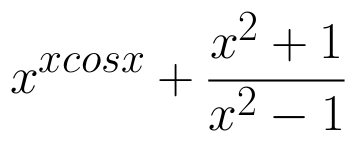. (View Answer Video)

Question 2 : Findfor the function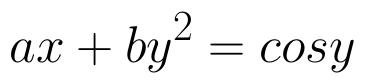. (View Answer Video)

Question 3 : Differentiate the function w.r.t.x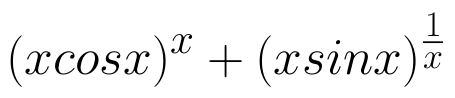. (View Answer Video)

Question 4 : If x and y are connected parametrically by the equation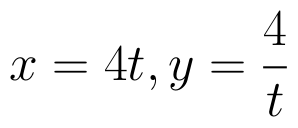, without eliminating the parameter, find. (View Answer Video)

Question 5 : Differentiate the function w.r.t.x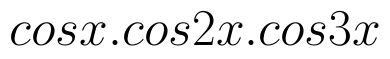. (View Answer Video)

### Integrals

Question 1 : Find :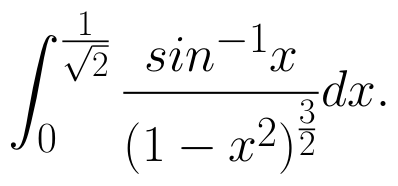(View Answer Video)

Question 2 : Find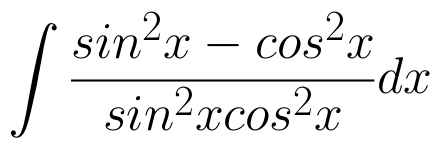. (View Answer Video)

Question 3 : Evaluate :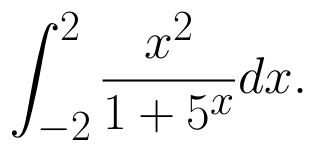(View Answer Video)

Question 4 : Evaluate :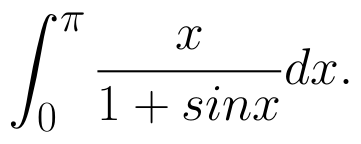(View Answer Video)

Question 5 : Evaluate :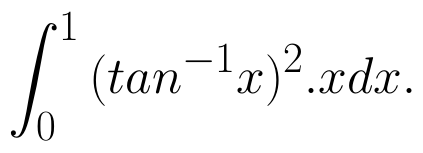(View Answer Video)

### Matrices

Question 1 : Compute: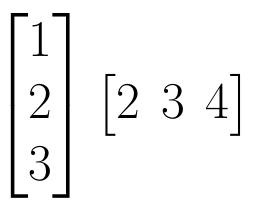. (View Answer Video)

Question 2 : Find the value of X, if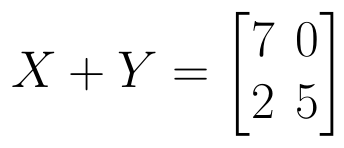and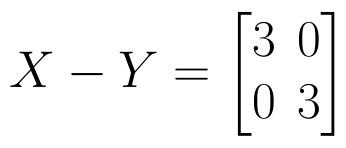. (View Answer Video)

Question 3 : Compute: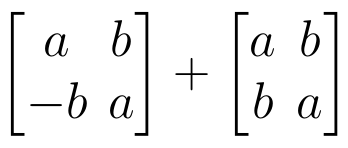. (View Answer Video)

Question 4 : Given,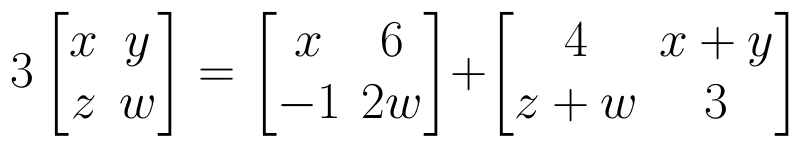, find the value of x. (View Answer Video)

Question 5 : Let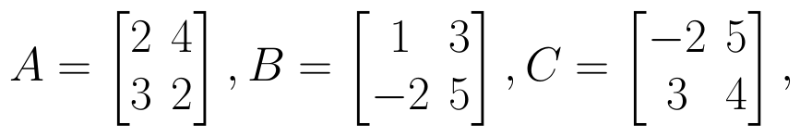Find AB. (View Answer Video)

### Vector Algebra

Question 1 : Find a vector in the direction of vector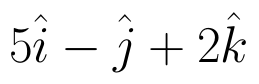which has magnitude 8 unit. (View Answer Video)

Question 2 : If the vertices A, B, C of a triangle ABC have position vectors (1, 2, 3), (-1, 0, 0), (0, 1, 2) respectively then find <ABC (<ABC is the angle between the vectors BA and BC). (View Answer Video)

Question 3 : Find |x|, if for a unit vector a,(x - a).(x + a) = 12. (View Answer Video)

Question 4 :  Find the sum of the vectors :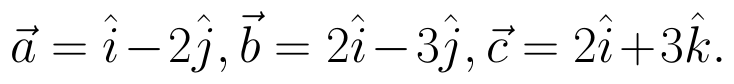(View Answer Video)

Question 5 : Find the direction of the vector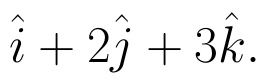(View Answer Video)